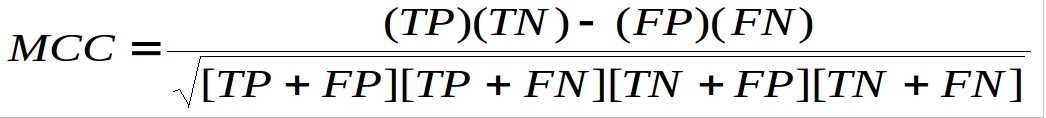# RNApin

In the development of RNApin web-server, first we have created RNA-208 dataset of 208 protein interacting RNA chains. We assigned the protein interacting and non-interacting nucleotides using 5.0 angstroms as a cut-off distance. Thereafter, we created sliding window patterns of different 3-25 length, where if the central nucleotide of window pattern was protein-interacting than whole pattern was used as positive otherwise used as negative pattern. To make fixed length window for the terminal nucleotides, we added dummy 'X' nucleotide at both the ends.

Binary profile of patterns (BPP)
The numerical representation of window patterns is necessary for the machine learning tools and Binary Profile of Patterns (BPP) based strategy is one of the widely adopted approach for the window-based machine learning. In BPP approach, we represented A, C, G, U and X nucleotides of the all window patterns in the binary form of {1,0,0,0,0}, {0,1,0,0,0}, {0,0,1,0,0}, {0,0,0,1,0} and {0,0,0,0,1} respectively. BPP generated five times higher input features than window size (e.g. 19-nucelotide long window pattern generates total 95 (19x5) input features). These binary representations of window pattern give information of nucleotide availability at specific position during machine learning based prediction model development.

Composition profile of patterns (CPP)
We used following three composition-based approaches-
Mono-nucleotide composition profile of patterns (MNCPP) - In MNCPP, we calculated mono-nucleotide composition of all nucleotides (A, C, G, U and X) for each window pattern separately. These five numerical values of composition were used as SVM an input.
Di-nucleotide composition profile of patterns (DNCPP) - In DNCPP, the di-nucleotide (AA, AC, AG, CG, AU,…, XX) composition of each window pattern calculated separately. It provided total 25 numerical values, which were used as SVM input. The DNCPP approach has advancement over MNCPP that it also provides information of neighboring nucleotides.
Tri-nucleotide composition profile of patterns (TNCPP) - In TNCPP, we calculated tri-nucleotide (AAA, AAC, AAG,…, XXX) composition of each window pattern separately. For each window pattern we found total 125 numerical values, which were used as SVM input features.

Support Vector Machine
In this study, a highly successful machine learning technique Support Vector Machine (SVM) was applied, which is based on the structural risk minimization principle of statistics learning theory. SVMs are a set of related supervised learning methods used for classification and regression mode (Vapnik, 1999). It has options of different parameters and kernels (e.g. Linear, polynomial, radial basis function and sigmoidal) to optimize according to need. We implemented SVMlight Version 6.02 package (Joachims, 1999) of SVM and machine learning. We applied various parameters and three different (linear, polynomial and radial basis function) kernels to develop our prediction models.

Five-fold Cross Validation
In this study, we used widely accepted five-fold cross-validation technique for training, testing and evaluation of our SVM prediction models. In this process, first we divided all positive and negative window patterns into five parts randomly. Each of these five sets consists of one-fifth of total positive and one-fifth of total negative window patterns. In five-fold cross validation technique, we used four sets as training and remaining one set as testing. This process was repeated five times in such a way that each set was used once as a test set. We calculated performance of each test set and overall performance of prediction model is an average performance of these five test sets.

Evaluation Methods
The prediction models were evaluated by five-fold cross validation techniques using the following formulas:-
Sensitivity = (TP / (TP+FN))*100

Specificity = (TN / (TN+FP))*100

Accuracy = (TP+TN / (TP+FP+TN+FN))*100Where TP and TN are correctly predicted Protein-Interacting Nucleotides (PINs) and non-PINs respectively. FP and FN are wrongly predicted PINs and non-PINs respectively.

Probability Score
RNApin predicts a probability score, which varies from 0-9 for each residue of protein sequence. At default 0.0 threshold, probability scores ranges between 0-4 and 5-9 predicted as non-interacting and interacting nucleotides respectively.